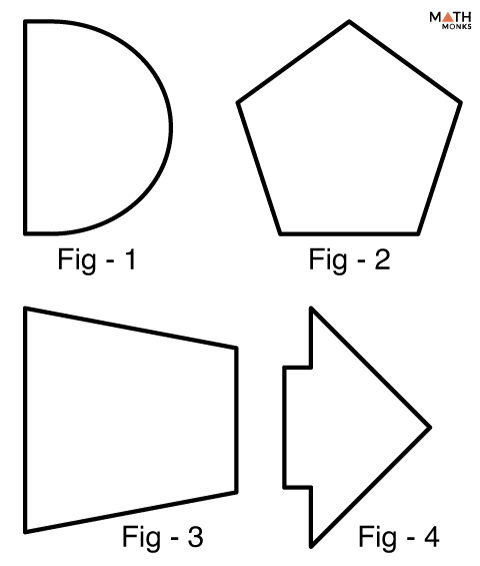# Reflection Symmetry

A shape is said to have a reflection symmetry if there exists at least one line that divides a figure into two halves such that one-half is the mirror image of the other half. Thus, it is also known as line symmetry or mirror symmetry. The line that divides the shape into two halves is the line of symmetry.

Not all shapes have lines of symmetry, or they may have several lines of symmetry. Shown below are some shapes having reflectional symmetry.

Let us consider the leaf above. When the line of symmetry passes through it, the leaf splits into two congruent halves. So, when we fold it along the dotted line, one half overlaps with the other.

The rectangle in figure-1 has 2 lines of symmetry. So it has 2-fold reflection symmetry. The square has 4 lines of symmetry. So it has 4-fold reflection symmetry. Likewise, X and H have 2-fold reflection symmetry, and the club shape has 1-fold reflection symmetry. Also, every regular polygon has reflectional symmetry.

Similarly, an equilateral triangle has 2-fold reflection symmetry and an isosceles triangle has 1-fold reflection symmetry.

But, which triangle has 0 reflection symmetries?

So, a scalene triangle, has no lines of symmetry, and thus has no reflection symmetries.

## Examples in Real-Life

1. Wings of a butterfly
2. The famous painting, Vitruvian Man by Leonardo da Vinci
3. Human face considering our nose as the line of symmetry

## Solved ExamplesWhich shape has reflectional symmetry in the diagram given alongside?

Solution:

The telephone in fig – 1 has reflection symmetry but the key in fig – 2 does not.Which figure in the diagram has a vertical line of reflection symmetry?

Solution:

Only the pentagon in fig – 2 has a vertical line of reflection symmetry. The rest have a horizontal line of reflection symmetry.

## FAQs

Q1. How many lines of reflection symmetry does the trapezoid have?

Ans. A trapezoid is not a symmetrical shape. So it does not have lines of reflection. However, an isosceles trapezoid has one vertical line of symmetry. So it has one line of reflection symmetry.

Q2. How many reflection symmetries does the regular hexagon have?

Ans. A regular hexagon has 3 reflection symmetries.

Q3. How many reflection symmetries does a regular decagon have?

Ans. A regular decagon10 reflection symmetries.

Q.4. Which quadrilateral will always have 4 fold reflectional symmetry?

Ans. A square has a 4 fold reflectional symmetry.